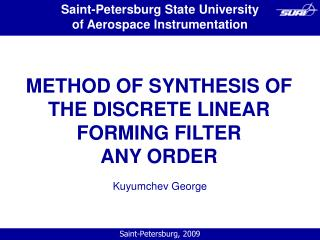DownloadDownload PresentationMETHOD OF SYNTHESIS OF THE DISCRETE LINEAR FORMING FILTER ANY ORDER

# METHOD OF SYNTHESIS OF THE DISCRETE LINEAR FORMING FILTER ANY ORDER

Download Presentation## METHOD OF SYNTHESIS OF THE DISCRETE LINEAR FORMING FILTER ANY ORDER

- - - - - - - - - - - - - - - - - - - - - - - - - - - E N D - - - - - - - - - - - - - - - - - - - - - - - - - - -
##### Presentation Transcript

1. Saint-Petersburg State University of Aerospace Instrumentation METHOD OF SYNTHESIS OF THE DISCRETE LINEAR FORMING FILTER ANY ORDER Kuyumchev George Saint-Petersburg, 2009

2. Introduction • The most comprehensible method, allowing to model processes in real time and without a methodical error, is the method of nonlinear functional transformation of normal random process. Mathematical expressions of algorithms of modelling, the following from it, allow to parallelize calculations at their realisation on multiprocessing COMPUTERS. • The basic disadvantage of a method is great size of the preparatory work, connected with calculation of parametres of algorithms. Thus, it is required to make decision in process of performance of numerical calculations for separate private problems that does practically impossible automation of process of synthesis of algorithm of modelling. • In this work some new results on synthesis of linear discrete filters of any order, which allow to receive the closed analytical expressions for calculation of coefficients of filters and by that, completely to automate the most labour-consuming part of a technique of synthesis of a method of nonlinear functional transformation, are presented.

3. SYNTHESIS OF THE DISCRETE LINEAR FORMING FILTER ANY ORDER • There are many different ways of synthesis of DFF on the set correlative-spectral characteristics of output signal. The main from these ways is synthesis of DFF on spectral density of a output signal by it factorization, however this method in practice can be applied successfully only for filters of the second order (theoretically for filters of 4th order) owing to the difficulties, arising at factorization of spectral density as function of pseudo-frequency. The methods of synthesis demanding the task of correlation function in several points, are developed, however these methods, first, lead to DFF of very high order, and secondly, they do not guarantee the behaviour of correlation function of modelled process out of an interval of the task of its correlation function. Here is offered other method of synthesis of DFF, materially, being intermediate between a statistical method and the methods of synthesis following from the theory of automatic control. • This technique is generalisation of techniques of synthesis of the similar filters, are received expressions for direct calculation of coefficients of the general model of autoregress-sliding of an average , and in the specified literature -only particular cases of synthesis : autoregression or a sliding average (the general method is only declared).

4. CONCLUSION • The method of calculation of coefficients of forming filters developed in work, allows to count coefficients for the general case of process of autoregress-sliding of an average, that minimises number of factors of the differential equation, realising this filter, and, hence, raises speed of algorithm of modelling. The received closed expressions for coefficients of filters allow to automate process of its synthesis.